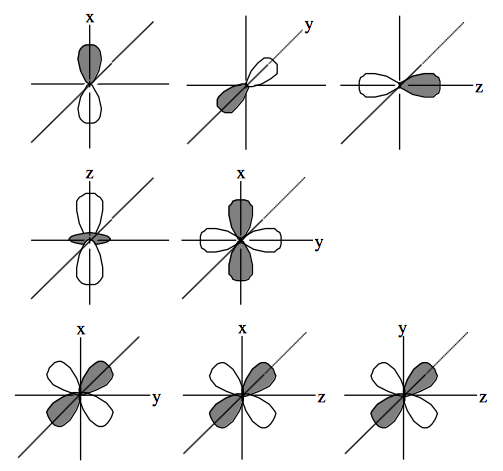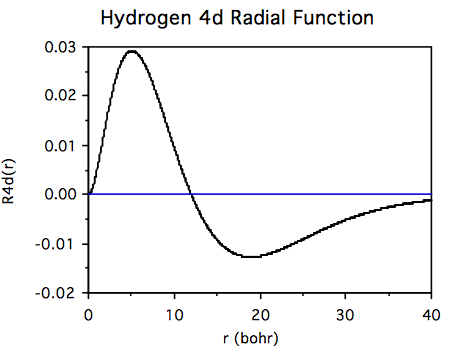22.2.4: iv. Review Exercises Solutions

•• Contributed by Jack Simons
• Professor Emeritus and Henry Eyring Scientist (Chemistry) at University of Utah

1.2. i. In ammonia the only "core" orbital is the N 1s and this becomes an a1 orbital in $$C_{3v}$$ symmetry. The N 2s orbitals and 3 H 1s orbitals become 2 $$a_1$$ and an e set of orbitals. The remaining N 2p orbitals also become 1 $$a_1$$ and a set of e orbitals. The total valence orbitals in $$C_{3v}$$ symmetry are $$3a_1$$ and 2e orbitals.

ii. In water the only core orbital is the O 1s and this becomes an a1 orbital in $$C_{2v}$$ symmetry. Placing the molecule in the yz plane allows us to further analyze the remaining valence orbitals as: O $$2p_z = a_1$$, O $$2p_y\text{ as } b_2\text{ , and O } 2p_x\text{ as } b_1$$. The H 1s + H 1s combination is an $$a_1$$ whereas the H 1s - H 1s combination is a $$b_2$$.

iii. Placing the oxygens of $$H_2O_2$$ in the yz plane (z bisecting the oxygens) and the (cis) hydrogens distorted slightly in +x and -x directions allows us to analyze the orbitals as follows. The core O 1s + O 1s combination is an a orbital whereas the O 1s - O 1s combination is aborbital. The valence orbitals are: O2s+O2s=a, O2s-O2s=b, O $$2p_x\text{ + O }2p_x$$ = b, O 2px - O 2px = a, O 2py + O 2py = a, O 2py - O 2py = b, O 2pz + O 2pz = b, O 2pz - O 2pz = a, H 1s + H 1s = a, and finally the H 1s - H 1s = b.

iv. For the next two problems we will use the convention of choosing the z axis as principal axis for the $$D_{\infty h}\text{, }D_{2h}\text{, and } C_{2v}$$ point groups and the xy plane as the horizontal reflection plane in $$C_s$$ symmetry.

\begin{align} & & D_{\infty h} & & D_{2h} & & C_{2v} & & C_s & \\ & N 1s & & \sigma_g & & a_g & & a_1 & & a^{\prime} & \\ & N 2s & & \sigma_g & & a_g & & a_1 & & a^{\prime} & \\ & N 2p_x & & \pi_{xu} & & b_{3u} & & b_1 & & a^{\prime} & \\ & N 2p_y & & \pi_{yu} & & b_{2u} & & b_2 & & a^{\prime} & \\ & N 2p_z & & \sigma_{u} & & b_{1u} & & a_1 & & a^{\prime\prime} & \end{align}

v. The Nitrogen molecule is in the yz plane for all point groups except the Cs in which case it is placed in the xy plane.

\begin{align} & & D_{\infty h} & & D_{2h} & & C_{2v} & & C_s & \\ & N 1s + N 1s & & \sigma_g & & a_g & & a_1 & & a^{\prime} & \\ & N 1s - N 1s & & \sigma_u & & b_{1u} & & b_2 & & a^{\prime} & \\ & N 2s + N 2s & & \sigma_g & & a_g & & a_1 & & a^{\prime} & \\ & N 2s - N 2s & & \sigma_u & & b_{1u} & & b_2 & & a^{\prime} & \\ & N 2p_x + N 2p_x & & \pi_{xu} & & b_{3u} & & b_1 & & a^{\prime} & \\ & N 2p_x - N 2p_x & & \pi_{xg} & & b_{2g} & & a_2 & & a^{\prime} & \\ & N 2p_y + N 2p_y & & \pi_{yu} & & b_{2u} & & a_1 & & a^{\prime} & \\ & N 2p_y - N 2p_y & & \pi_{yg} & & b_{3g} & & b_2 & & a^{\prime} & \\ & N 2p_z + N 2p_z & & \sigma_{u} & & b_{1u} & & b_2 & & a^{\prime\prime} & \\ & N 2p_z - N 2p_z & & \sigma_{g} & & a_{g} & & a_1 & & a^{\prime\prime} & \end{align}

3.4.